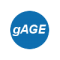If you wish to contribute or participate in the discussions about articles you are invited to contact the Editor

# Precise modelling terms for PPPFundamentals
Title Precise modelling terms for PPP
Author(s) J. Sanz Subirana, J.M. Juan Zornoza and M. Hernández-Pajares, Technical University of Catalonia, Spain.
Year of Publication 2011The Precise Point Positioning (PPP) technique allows achieving centimetre level accuracy for static positioning and decimetre level for kinematic, typically, after the best part of one hour. This high accuracy requires an accurate measurements modelling, where all model terms must be taken into account (up to the centimetre level or better).

This modelling involves the following terms, among those considered in Code Based Positioning:

• Precise satellite orbits and clocks:
The precise orbits and clocks files (see Precise GNSS Satellite Coordinates Computation) must be used instead of the broadcast ones used in conventional Code Based Positioning.
The satellite coordinates between epochs can be computed by polynomial interpolation.
As an example of polynomial interpolation, the Lagrange method is presented as follows. Given a table of values $\displaystyle{ (x_i,y_i), i=1,…,n, }$ the interpolated value $\displaystyle{ y≃P_n(x) }$ at a given $\displaystyle{ x }$ can be computed as (see Precise GNSS Satellite Coordinates Computation):
$\displaystyle{ \begin{array}{lll} P_n(x)&=& \sum_{i=1}^n{y_i \frac{\prod_{j\neq i}{(x-x_j)}}{\prod_{j\neq i}{(x_i-x_j)}}}=\\ &=& y_1 \frac{x-x_2}{(x_1-x_2)} \cdots \frac{x-x_n}{(x_1-x_n)}+\cdots\\ & &+y_i \frac{x-x_1}{(x_i-x_1)} \cdots \frac{x-x_{i-1}}{(x_i-x_{i-1})}\frac{x-x_{i+1}}{(x_i-x_{i+1})}\cdots \frac{x-x_n}{(x_i-x_n)}+\cdots\\ & & +y_n \frac{x-x_1}{(x_n-x_1)} \cdots\frac{x-x_{n-1}}{(x_n-x_{n-1})} \end{array} \qquad \mbox{(1)} }$

Notice that, the orbits are referred to the satellite mass centre, and thence, the Satellite Antenna Phase Centre offset must be applied to compute the $\displaystyle{ {\boldsymbol \Delta}_{APC} }$ vector offset (see Satellite Antenna Phase Centre).
$\displaystyle{ {\mathbf r}_{sat_{_{APC}}}={\mathbf r}_{sat_{_{MC}}}+{\mathbf R}\cdot {\boldsymbol \Delta}_{_{APC}} \qquad \mbox{(2)} }$

The satellite clocks should not be interpolated and thence, only epochs having clocks available must be used (Precise GNSS Satellite Coordinates Computation).

Relativistic effects(see  for a very good review of relativistic effects on GPS): The gravitational path range correction (3) (see Relativistic Path Range Effect), can be added among the satellite clock correction due to the orbit eccentricity (4) considered in Code Based Positioning (see Relativistic Clock Correction).
$\displaystyle{ \Delta \rho_{_{rel}}=\frac{2\,\mu}{c^2}ln\frac{r^{sat}+r_{rcv}+r_{rcv}^{sat}}{r^{sat}+r_{rcv}-r_{rcv}^{sat}} \qquad \mbox{(3)} }$
$\displaystyle{ \Delta_{rel}=- 2\, \frac{\mathbf{r} \cdot \mathbf{v}}{c^2} \qquad \mbox{(4)} }$

• Atmospheric effects:
The ionospheric refraction and DCBs are removed using the ionosphere free combination of measurements (see details in Ionosphere-free Combination for Dual Frequency Receivers).

The tropospheric refraction can be modelled by (5) (see Tropospheric Delay )
$\displaystyle{ T(E)=T_{z,dry}\cdot M_{dry}(E)+T_{z,wet}\cdot M_{wet}(E) \qquad \mbox{(5)} }$

where the mapping of Niell is used. The dry and wet tropospheric delays are given by
$\displaystyle{ \begin{array}{l} T_{z,dry}= a\; e^{-b\; H}\\ T_{z,wet}= T_{z_0,wet}+\Delta T_{z,wet} \qquad \mbox{(6)} \end{array} }$

The deviation of the zenith tropospheric delay $\displaystyle{ \Delta T_{z,wet} }$ regarding to the nominal $\displaystyle{ T_{z_0,wet} }$ must be estimated in the Kalman filter, together with the coordinates, clock and carrier phase biases.

• Antenna biases and orientation:
The satellite and receiver antenna phase centres can be found in the IGS ANTEX files, after the GPS week 1400 (see Antenna Phase Centre).

The carrier phase wind-up effect due to the satellite motion is given by (7) (see Carrier Phase Wind-up Effect)
$\displaystyle{ \Delta \phi=2N\pi+sign(\zeta)\cdot \arccos \left( \frac{\vec{D}^{\prime}\cdot\vec{D}}{\|\vec{D}^{\prime}\|\cdot\|\vec{D}\|}\right) \qquad \mbox{(7)} }$

The satellites under eclipse conditions should be removed from the computation due to the largest orbit error. The eclipse condition is given by (8) (see Satellite Eclipses)
$\displaystyle{ \cos \phi = \frac{{\mathbf r}_{sat}\cdot {\mathbf r}_{sun}}{|{\mathbf r}_{sat}\cdot {\mathbf r}_{sun}|} \lt 0 \qquad \mbox{and} \qquad r_{sat} \sqrt{1-\cos^2 \phi}\, \lt a_e \qquad \mbox{(8)} }$

• Earth deformation effects:
Solid tides can be modelled by equations (9) and (10) (see Solid Tides )
$\displaystyle{ \Delta {\mathbf r}= \sum_{j=2}^{3}{\frac{G\,M_j\,R_e^4}{G\,M_\oplus\,R_j^3}} \left \{h_2 \,\hat{\mathbf r} \left ( \frac{3}{2} (\hat{\mathbf R}_j \cdot \hat{\mathbf r})^2 -\frac{1}{2}\right) + 3\,l_2\,(\hat{\mathbf R}_j \cdot \hat{\mathbf r}) \left [\hat{\mathbf R}_j-(\hat{\mathbf R}_j \cdot \hat{\mathbf r})\,\hat{\mathbf r} \right ]\right \} \qquad \mbox{(9)} }$
$\displaystyle{ \Delta {\mathbf r}= \sum_{j=2}^{3}{\frac{G\,M_j\,R_e^5}{G\,M_\oplus\,R_j^4}} \left \{h_3 \,\hat{\mathbf r} \left ( \frac{5}{2} (\hat{\mathbf R}_j \cdot \hat{\mathbf r})^3 -\frac{3}{2} (\hat{\mathbf R}_j \cdot \hat{\mathbf r})\right) + l_3\,\left (\frac{15}{2} (\hat{\mathbf R}_j \cdot \hat{\mathbf r})^2 - \frac{3}{2} \right ) \left [\hat{\mathbf R}_j-(\hat{\mathbf R}_j \cdot \hat{\mathbf r})\,\hat{\mathbf r} \right ]\right \} \qquad \mbox{(10)} }$

Ocean loading and Pole Tides are second order effects and can be neglected for PPP accuracies at the centimetre level (see comments in Ocean loading and Pole Tide).